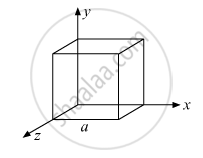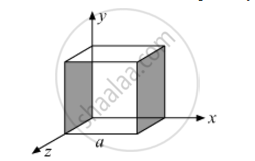# Given the Electric Field in the Region E=2xi,Find the Net Electric Flux Through the Cube and the Charge Enclosed by It. - Physics

Given the electric field in the region vecE=2xhati, find the net electric flux through the cube and the charge enclosed by it.#### Solution

Since the electric field has only x component, for faces normal to x direction, the angle between E and S is ±π/2. Therefore, the flux is separately zero for each face of the cube except the two shaded ones.The magnitude of the electric field at the left face is EL = 0      (As x = 0 at the left face)

The magnitude of the electric field at the right face is ER = 2a    (As x = a at the right face)

The corresponding fluxes are

phi_L=vecE.DeltavecS=0

phi_R=vecE_R.DeltavecS=E_RDeltaScostheta=E_RDeltaS " "(.:theta=0^@)

ϕR= ERa2

Net flux (ϕ) through the cube = ϕL+ϕR=0+ERa2=ERa2

ϕ=2a(a)2=2a3

We can use Gauss’s law to find the total charge q inside the cube.

phi=q/(epsilon_0)

q=ϕε0=2a3ε0

Concept: Electric Flux
Is there an error in this question or solution?